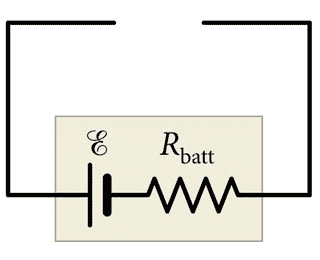# Length and Area of Cylindrical Nichrome Resistor

## Homework Statement

You must complete the circuit of (Figure 1) in such a way that it draws a current of 0.450 A from the battery. The battery maintains a potential difference of 10.0 V with no load, but has an internal resistance of Rbatt = 15.0 Ω . The only material you have is 20.0 mm^3 of nichrome, and you must use all of it. your plan is to form the nichrome into a cylindrical resistor. [The conductivity of nichrome is 6.7 × 105 A/(V⋅m).]Part A
What must the cylinder length be?

Part B

What must the cylinder cross-sectional area be?

I = V/R
R = L/(A*σ)
A = πr^2

## The Attempt at a Solution

First, I solved for the resistance the created resistor must have. Since the two resistors would be in series, I solved the equivalent resistance to be R = Rn + Rb. (Rn = Resistance of new resistor that is being solved for, Rb = resistance of battery). To draw the correct amount of current out of the battery, I = V/(Rn+Rb). Solving for Rn, I got Rn = V/I-Rb = 10/0.45 - 15 = 7.22 Ω.

I'm pretty sure I need to use this equation: R = L/(A*σ) to solve for L and A, but since I only know R and σ, I still have two unknown variables to solve for, and only one equation. I'm guessing I need to incorporate the given detail that there is only 20 mm^3 of nichrome wire available, but I am not sure how to do that. Is there another equation I should use to do so, like volume or surface area?

## Answers and Replies

Is there a way to get volume out of L and A?

Edit: P.S. Welcome to Physics Forums.

gneill
Mentor
Hi Robotic-Cow,

Welcome to Physics Forums!

First, I solved for the resistance the created resistor must have. Since the two resistors would be in series, I solved the equivalent resistance to be R = Rn + Rb. (Rn = Resistance of new resistor that is being solved for, Rb = resistance of battery). To draw the correct amount of current out of the battery, I = V/(Rn+Rb). Solving for Rn, I got Rn = V/I-Rb = 10/0.45 - 15 = 7.22 Ω.
Looks good so far.
I'm pretty sure I need to use this equation: R = L/(A*σ) to solve for L and A, but since I only know R and σ, I still have two unknown variables to solve for, and only one equation. I'm guessing I need to incorporate the given detail that there is only 20 mm^3 of nichrome wire available, but I am not sure how to do that. Is there another equation I should use to do so, like volume or surface area?
Yup, you're given a volume value so an equation for the volume of the resistor you're creating seems appropriate.

•Robotic-Cow
Thanks so much for the help! I managed to solve both length and cross-sectional area by rearranging the volume equation in terms of radius, and plugging it back into the equation for resistance to solve for length.